Skip to main content

Mathematics

# Mathematics

BSc
• UCAS code G100
• Option 3 years full time
• Year of entry 2021

## The course

Mathematics is in everything: we use it in every aspect of our lives – from managing household finances and investments to working at the cutting edge of digital communications, engineering, aviation, satellite navigation, medical science, weather forecasting, psychology, sociology, insurance, or the financial markets of the global economy. It is one of the oldest academic disciplines and yet it sits at the heart of our 21st century lives.

Our flagship BSc course brings the beauty and breadth of mathematics to life, inviting you to delve deep into the world of abstract structures and ideas, whilst also equipping you with the practical skills and experience that will set you apart in the world of work. Guided by experts in the field, you will receive a thorough grounding in the key methods and concepts that underpin our subject, with the flexibility to tailor your studies in years 2 and 3, thanks to the courses modular structure.

Our broad curriculum is influenced by the department’s world-class research activities. We are renowned for our work in pure mathematics, information security, statistics and theoretical physics, and our BSc course spans pure and applied mathematics, statistical analysis, financial mathematics, the mathematics of information, and more. We also offer an array of postgraduate opportunities, and provided you make good progress in year 1 you will have the option of transferring onto our four-year masters courses to help take your mathematics to a higher level, particularly if you want to pursue a career in industry or research. It might also be possible to transfer sideways into the second year of one of our other undergraduate mathematics courses.

Join our friendly and inspiring department and you will benefit from a thoroughly supportive learning environment. We offer small group tutorials, problem-solving sessions, practical workshops and IT classes, as well as generous staff office hours and a dedicated personal adviser to guide you through your studies. We also offer CV writing workshops and a competitive work placement scheme. Our graduates are in great demand for their numeracy, analytical skills, data handling powers, logical thinking and creative problem-solving abilities.

• Feel at home in a friendly department with a strong focus on small group teaching, where you will be known as an individual.
• Enjoy a varied and flexible curriculum, and tailor your degree to your own interests.

#### Core Modules

Year 1
• In this module you will develop an understanding of how mathematics has been used to describe space over the last 2,500 years. You will look at ruler and compass constructions from ancient Greece, the influence of algebra on geometry in the renaissance, and the intricate and beautiful fractal patterns developed by Benoît Mandelbrot in the 1970s. You will learn to sketch simple curves using polar coordinates, draw and classify conics, and use simple arguments to distinguish between countable and uncountable sets.

• In this module, you will develop an understanding of how the techniques for solving differential equations can be applied to describe the real world. You will look at situations from balls flying through the air to planets orbiting the stars, including why the moon continues to orbit the Earth and not the Sun. You will consider the chatotic motion of a pendulum, and examine Einstein's theory of special relativity to describe the propagation of matter and light at high speeds.

• In this module you will develop an understanding of the notion of probability and the basic theory and methods of statistics. You will look at random variables and their distributions, calculate probabilities of events that arise from standard distributions, estimate means and variances, and carry out t tests for means and differences of means. You will also consider the notions of types of error, power and significance levels, gaining experience in sorting a variety of data sets in a scientific way.

• In this module, you will develop an understanding of the key concepts in Calculus, including differentiation and integration. You will learn how to factorise polynomials and separate rational functions into partial fractions, differentiate commonly occurring functions, and find definite and indefinite integrals of a variety of functions using substitution or integration by parts. You will also examine how to recognise the standard forms of first-order differential equations, and reduce other equations to these forms and solve them.

• In this module you will develop an understanding of the calculus functions of more than one variable and how it may be used in areas such as geometry and optimisation. You learn how to manipulate partial derivatives, construct and manipulate line integrals, represent curves and surfaces in higher dimensions, calculate areas under a curve and volumes between surfaces, and evaluate double integrals, including the use of change of order of integration and change of coordinates.

• In this module you will develop an understanding of the fundamental algebraic structures, including familiar integers and polynomial rings. You will learn how to apply Euclid's algorithm to find the greatest common divisor of two integers, and use mathematical induction to prove simple results. You will examine the use of arithmetic operations on complex numbers, extract roots of complex numbers, prove De Morgan's laws, and determine whether a given mapping is bijective.

• In this module you will develop an understanding of basic linear algebra, in particular the use of matrices and vectors. You will look at the basic theoretical and computational techniques of matrix theory, examining the power of vector methods and how they may be used to describe three-dimensional space. You will consider the notions of field, vector space and subspace, and learn how to calculate the determinant of an n x n matrix.

• In this module you will develop an understanding of key mathematical concepts such as the construction of real numbers, limits and convergence of sequences, and continuity of functions. You will look at the infinite processes that are essential for the development of areas such as calculus, determining whether a given sequence tends to a limit, and finding the limits of sequences defined recursively.

Year 2
• In this module you will develop an understanding of vectors and matrices within the context of vector spaces, with a focus on deriving and using various decompositions of matrices, including eigenvalue decompositions and the so-called normal forms. You will learn how these abstract notions can be used to solve problems encountered in other fields of science and mathematics, such as optimisation theory.

• In this module you will develop an understanding of the basic complex variable theory. You will look at the definitions of continuity and differentiability of a complex valued function at a point, and how Cauchy-Riemann equations can be applied. You will examine how to use a power series to define the complex expontential function, and how to obtain Taylor series of rational and other functions of standard type, determining zeros and poles of given functions. You will also consider how to use Cauchy's Residue Theorem to evaulate real integrals.

• In this module you will develop an understanding of the convergence of series. You will look at the Weierstrass definition of a limit and use standard tests to investigate the convergence of commonly occuring series. You will consider the power series of standard functions, and analyse the Intermediate Value and Mean Value Theorems. You will also examine the properties of the Riemann integral.

• This course introduces you to the basics of Python programming for building solutions to mathematics-based tasks. This will encourage a deeper understanding of the mathematics that you will learn across your degree, developing general mathematical skills and group working. Additionally, the module will provide guidance through the process of applying for a summer internship or job, as well as reviewing the range of career options available to Mathematicians upon graduation.

Year 3
• All modules are optional

#### Optional Modules

There are a number of optional course modules available during your degree studies. The following is a selection of optional course modules that are likely to be available. Please note that although the College will keep changes to a minimum, new modules may be offered or existing modules may be withdrawn, for example, in response to a change in staff. Applicants will be informed if any significant changes need to be made.

Year 1
• All modules are core
Year 2
• In this module you will develop an understanding of the concepts of scalar and vector fields. You examine how vector calculus is used to define general coordinate systems and in differential geometry. You will learn how to solve simple partial differential equations by separating variables, and become familiar with how these concepts can be appield in the field of dynamics of inviscid fluids.

• In this module you will develop an understanding of statistical modelling, becoming familiar with the theory and the application of linear models. You will learn how to use the classic simple linear regression model and its generalisations for modelling dependence between variables. You will examine how to apply non-parametric methods, such as the Wilxocon and Kolmogorov-Smirnov goodness-of-fit tests, and learn to use the R open source software package.

• In this module you will develop an understanding of the basic principles of the mathematical theory of probability. You will use the fundamental laws of probability to solve a range of problems, and prove simple theorems involving discrete and continuous random variables. You will learn how to forumulate an explain fundamental limit theorems, such as the weak law of large numbers and the central limit theorem.

• In this module you will develop an understanding of the basic concepts of graph theory and linear programming. You will consider how railroad networks, electrical networks, social networks, and the web can be modelled by graphs, and look at basic examples of graph classes such as paths, cycles and trees. You will examine the flows in networks and how these are related to linear programming, solving problems using the simplex algorithm and the strong duality theorem.

• In this module you will develop an understanding of the concepts arising when the boundary conditions of a differential equation involves two points. You will look at eigenvalues and eigenfunctions in trigonometric differential equations, and determine the Fourier series for a periodic function. You will learn how to manipulate the Dirac delta-function and apply the Fourier transform. You will also examine how to solve differential equations where the coefficients are variable.

• In this module you will develop an understanding of ring theory and how this area of algebra can be used to address the problem of factorising integers into primes. You will look at how these ideas can be extended to develop notions of 'prime factorisation' for other mathematical objects, such as polynomials. You will investigate the structure of explicit rings and learn how to recognise and construct ring homomorphisms and quotients. You will examine the Gaussian integers as an example of a Euclidean ring, Kronecker's theorem on field extensions, and the Chinese Remainder Theorem.

• In this module you will develop an understanding of the algebraic structures known as groups. You will look at how groups represent symmetries in the world around us, examining examples that arise from the theory of matrices and permutations. You will see how groups are ubiquitous and used in many different fields of human study, including mathematics, physics, the study of crystals and atoms, public key cryptography, and music theory. You also will also consider how various counting problems concerning discrete patterns can be solved by means of group actions.

• In this module you will develop an understanding of the language and concepts of linear algebra that are used within Mathematics. You will look at topics in linear algebra and the theory of modules, which can be seen as generalisations of vector spaces. You will learn how to use alternative matrix representations, such as the Jordan canonical or the rational canonical form, and see why they are important in mathematics.

Year 3
• You will carry out a detailed investigation on a topic of your choosing, guided by an academic supervisor. You will prepare a written report around 7,000 words in length, and give a ten-minute presentation outlining your findings.

• In this module you will develop an understanding of a range of methods for teaching children up to A-level standard. You will act act as a role model for pupils, devising appropriate ways to convey the principles and concepts of mathematics. You will spend one session a week in a local school, taking responsibility for preparing lesson plans, putting together relevant learning aids, and delivering some of the classes. You will work with a specific teacher, who will act as a trainer and mentor, gaining valuable transferable skills.

• In this module you will develop an understanding of how prime numbers are the building blocks of the integers 0, ±1, ±2, … You will look at how simple equations using integers can be solved, and examine whether a number like 2017 should be written as a sum of two integer squares. You will also see how Number Theory can be used in other areas such as Cryptography, Computer Science and Field Theory.

• In this module you will develop an understanding of a range methods used for testing and proving primality, and for the factorisation of composite integers. You will look at the theory of binary quadratic forms, elliptic curves, and quadratic number fields, considering the principles behind state-of-the art factorisation methods. You will also look at how to analyse the complexity of fundamental number-theoretic algorithms.

• In this module you will develop an understanding the different classes of computational complexity. You will look at computational hardness, learning how to deduce cryptographic properties of related algorithms and protocols. You will examine the concept of a Turing machine, and consider the millennium problems, including P vs NP, with a \$1,000,000 prize on offer from the Clay Mathematics Institute if a correct solution can be found.

• In this module you will develop an understanding of efficient algorithm design and its importance for handling large inputs. You will look at how computers have changed the world in the last few decades, and examine the mathematical concepts that have driven these changes. You will consider the theory of algorithm design, including dynamic programming, handling recurrences, worst-case analysis, and basic data structures such as arrays, stacks, balanced search trees, and hashing.

• In this module you will develop an understanding of quantum theory, and the development of the field to explain the behaviour of particles at the atomic level. You will look at the mathematical foundations of the theory, including the Schrodinger equation. You will examine how the theory is applied to one and three dimensional systems, including the hydrogen atom, and see how a probabilistic theory is required to interpret what is measured.

• In this module you will develop an understanding of how the Rayleigh-Ritz variational principle and perturbation theory can be used to obtain approximate solutions of the Schrödinger equation. You will look at the mathematical basis of the Period Table of Elements, considering spin and the Pauli exclusion principle. You will also examine the quantum theory of the interaction of electromagnetic radiation with matter.

• In this module you will develop an understanding of how the theory of ideal fluids can be used to explain everyday phenomena in the world around us, such as how sound travels, how waves travel over the surface of a lake, and why golden syrup (or volcanic lava) flows differently from water. You will look at the essential features of compressible flow and consider basic vector analysis techniques.

• In this module, you will develop an understanding of non-linear dynamical systems. You will investigate whether the behaviour of a non-linear system can be predicted from the corresponding linear system, and see how dynamical systems can be used to analyse mechanisms such as the spread of disease, the stability of the universe, and the evolution of economic systems. You will gain an insight into the 'secrets' of the non-linear world and the appearance of chaos, examining the significant developments achieved in this field during the final quarter of the 20th Century.

• In this module you will develop an understanding of the mathematics of communication, focusing on digital communication as used across the internet and by mobile telephones. You looking at compression, considering how small a file, such as a photo or video, can be made, and therefore how the use of data can be minimised. You will examine error correction, seeing how communications may be correctly received even if something goes wrong during the transmission, such as intermittent wifi signal. You will also analyse the noiseless coding theorem, defining and using the concept of channel capacity.

• In this module you will develop an understanding of how the behaviour of quantum systems can be harnessed to perform information processing tasks that are otherwise difficult, or impossible, to carry out. You will look at basic phenomena such as quantum entanglement and the no-cloning principle, seeing how these can be used to perform, for example, quantum key distribution. You will also examine a number of basic quantum computing algorithms, observing how they outperform their classical counterparts when run on a quantum computer.

• In this module you will develop an understanding of how financial markets operate, with a focus on the ideas of risk and return and how they can be measured. You will look at the random behaviour of the stock market, Markowitz portfolio optimisation theory, the Capital Asset Pricing Model, the Binomial model, and the Black-Scholes formula for the pricing of options.

• In this module you will develop an understanding of the role of mathematics and statistics in securities markets. You will investigate the validity of various linear and non-linear time series occurring in finance, and apply stochastic calculus, including partial differential equations, for interest rate and credit analysis. You will also consider how spot rates and prices for Asian and barrier exotic options are modelled.

• In this module you will develop an understanding of some of the standard techniques and concepts of combinatorics, including methods of counting, generating functions, probabilistic methods, permutations, and Ramsey theory. You will see how algebra and probability can be used to count abstract mathematical objects, and how to calculate sets by inclusion and exclusion. You will examine the applications of number theory and consider the use of simple probabilistic tools for solving combinatorial problems.

• In this module you will develop an understanding of how error correcting codes are used to store and transmit information in technologies such as DVDs, telecommunication networks and digital television. You will look at the methods of elementary enumeration, linear algebra and finite fields, and consider the main coding theory problem. You will see how error correcting codes can be used to reconstruct the original information even if it has been altered or degraded.

• In this module you will develop an understanding of how error correcting codes are used to store and transmit information in technologies such as DVDs, telecommunication networks and digital television. You will look at the methods of elementary enumeration, linear algebra and finite fields, and consider the main coding theory problem. You will see how error correcting codes can be used to reconstruct the original information even if it has been altered or degraded.

• In this module you will develop an understanding of public key cryptography and the mathematical ideas that underpin it, including discrete logarithms, lattices and elliptic curves. You will look at several important public key cryptosystems, including RSA, Rabin, ElGamal encryption and Schnorr signatures. You will consider notions of security and attack models relevant for modern theoretical cryptography, such as indistinguishability and adaptive chosen ciphertext attack.

• In this module you will develop an understanding of Field Theory. You will learn how to express equations such as X2017=1 in a formal algebraic setting, how to classify finite fields, and how to determine the number of irreducible polynomials over a finitie field. You will also consider some the applications of fields, including ruler and compass constructions and why it is impossible to generically trisect an angle using them.

• In this module you will develop an understanding of the algebraic structures known as groups. You will look at how groups represent symmetries in the world around us, examining examples that arise from the theory of matrices and permutations. You will see how groups are ubiquitous and used in many different fields of human study, including mathematics, physics, the study of crystals and atoms, public key cryptography, and music theory. You also will also consider how various counting problems concerning discrete patterns can be solved by means of group actions.

• In this module you will develop an understanding of the language and concepts of linear algebra that are used within Mathematics. You will look at topics in linear algebra and the theory of modules, which can be seen as generalisations of vector spaces. You will learn how to use alternative matrix representations, such as the Jordan canonical or the rational canonical form, and see why they are important in mathematics.

• In this module you will develop an understanding of geometric objects and their properties. You will look at objects that are preserved under continuous deformation, such as through stretching or twisting, and will examine knots and surfaces. You will see how colouring a knot can be used to determine whether or not it can be transformed into the unknot without any threading. You will also consider why topologists do not distinguish between a cup and a donut.

The course has a flexible, modular structure and you will take a total of 12 course units at a rate of four, 30-credit modules per year. In addition to our compulsory core modules, you will be free to choose between a number of optional courses. Some contribute 15 credits to your overall award while others contribute the full 30.

We use a variety of teaching methods and there is a strong focus on small group teaching in the department. You will attend 12 to 15 hours of formal teaching in a typical week, including lectures, tutorials, problem-solving workshops and practical sessions in statistics and computational mathematics. You will also be expected to work on worksheets, revision and project work outside of these times. In year 2, teaching will mainly be delivered through lectures and workshops and in year 3, mostly through relatively small group lectures.

Our courses are mostly examined by written exams taken in the summer term, but some of our statistics and computational courses also have project components and between 10% and 30% of your final mark for each core module in year 1 will come from coursework. Some of the first year modules also include tests that contribute 10% of the final mark. In the first term of year 2 you will work in small groups to prepare a report and an oral presentation on a mathematical topic of your choice, which will contribute to one of your core module grades. CV writing skills are also embedded into that course. In year 3 there are two optional courses which are examined solely by a project and presentation.

2nd in the UK for Research Impact

Source: THE REF institutions ranked by subject, 2014

98% overall satisfaction from our students

Source: NSS, 2017

96% of our graduates go on to work or further study within six months of graduating

Source: DLHE, 2017

Browse all

## Explore Royal HollowayAll undergraduates starting with us in 2020 onwards have the opportunity to take a Placement Year, which will add even more value to your studies.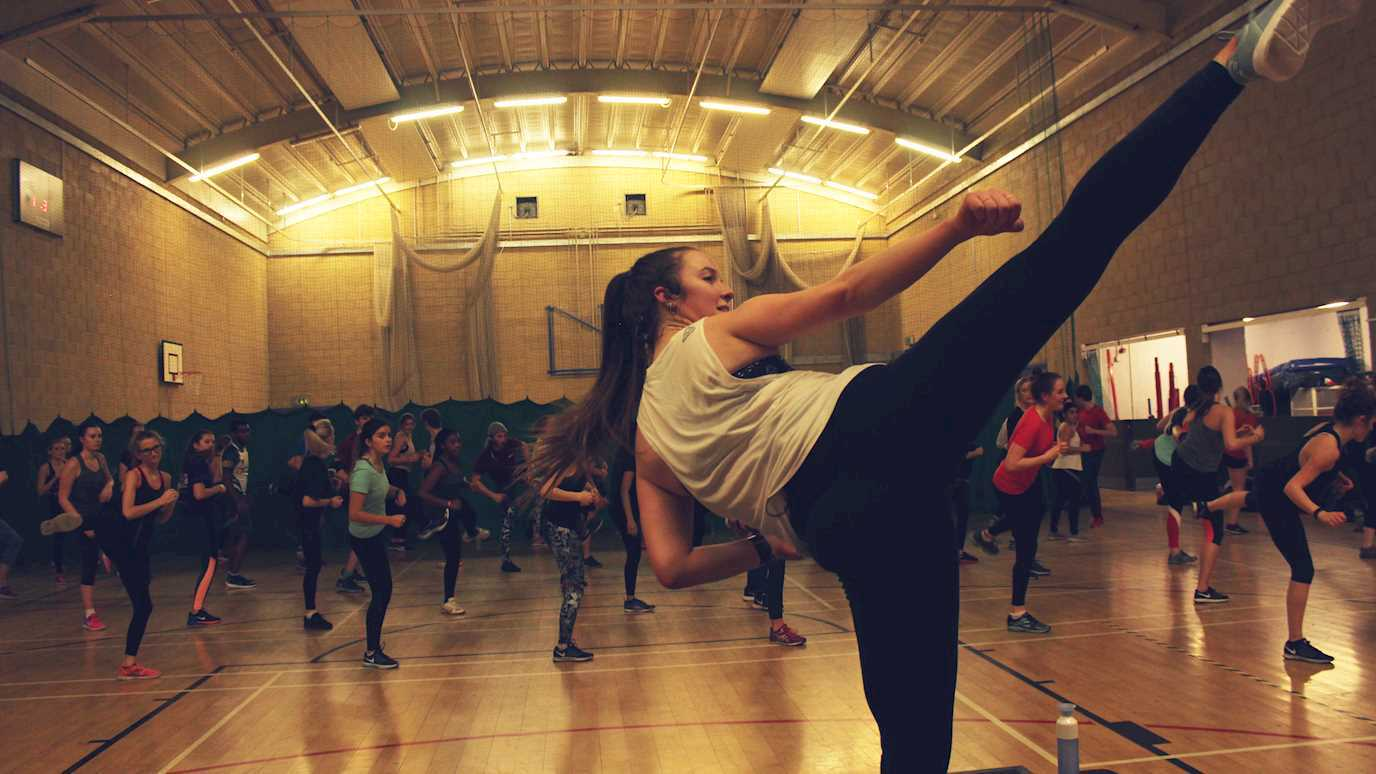There are lots of exciting ways to get involved at Royal Holloway. Discover new interests and enjoy existing ones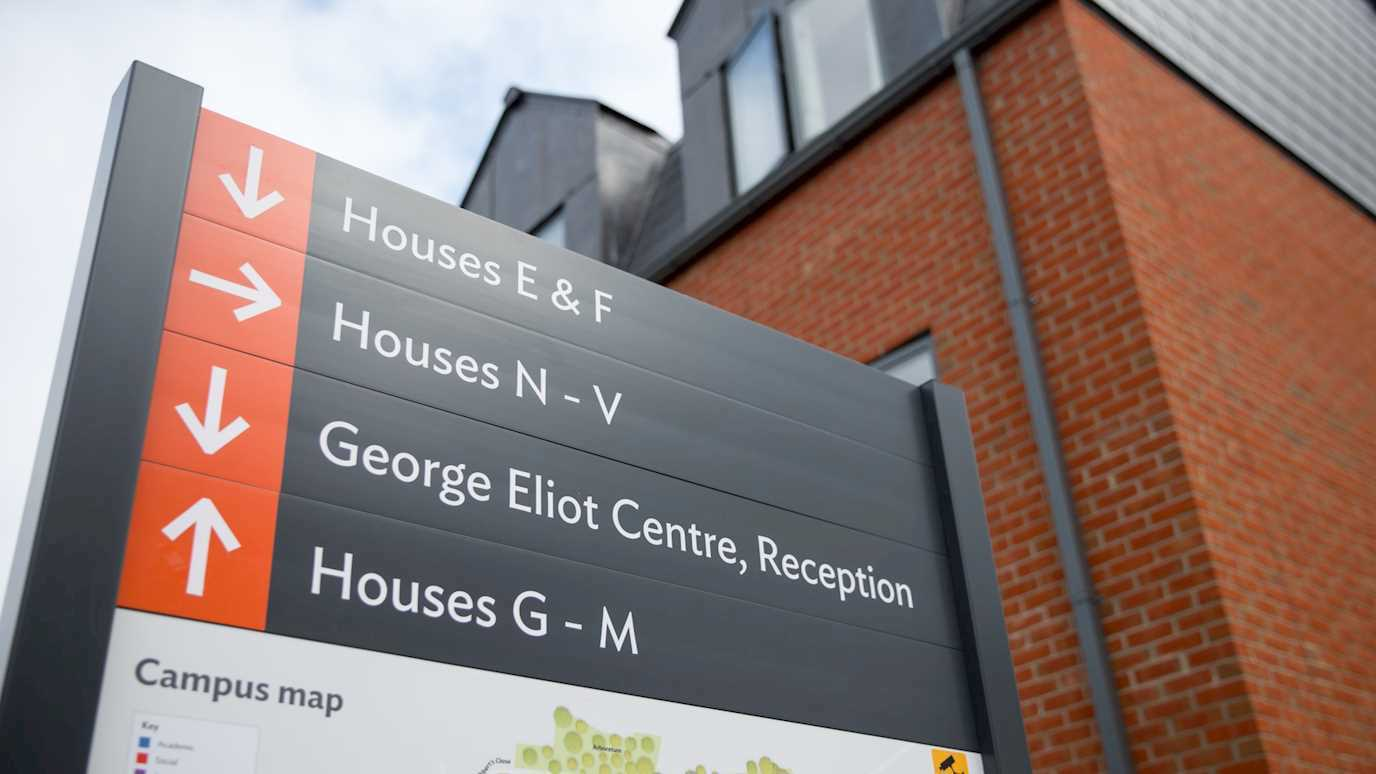Heading to university is exciting. Finding the right place to live will get you off to a good start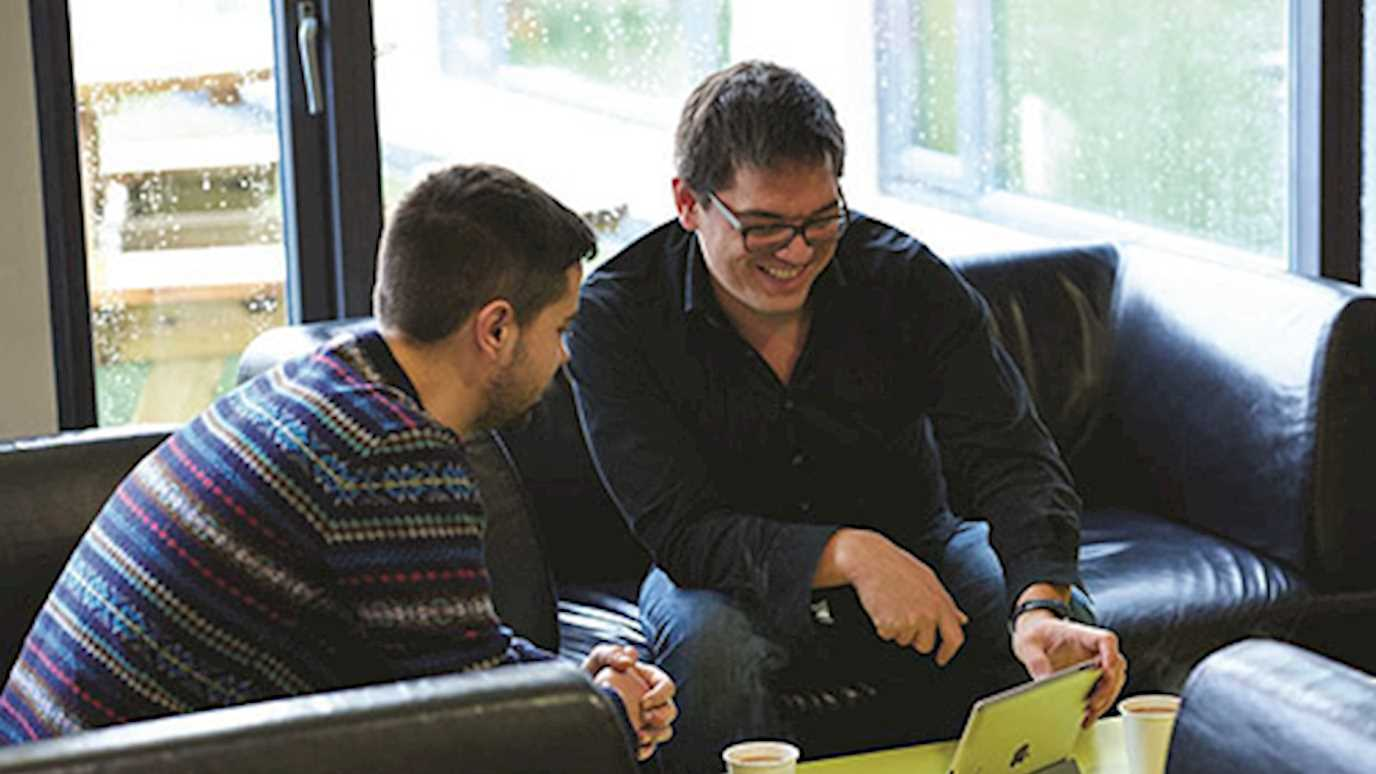Whether you need support with your health or practical advice on budgeting or finding part-time work, we can help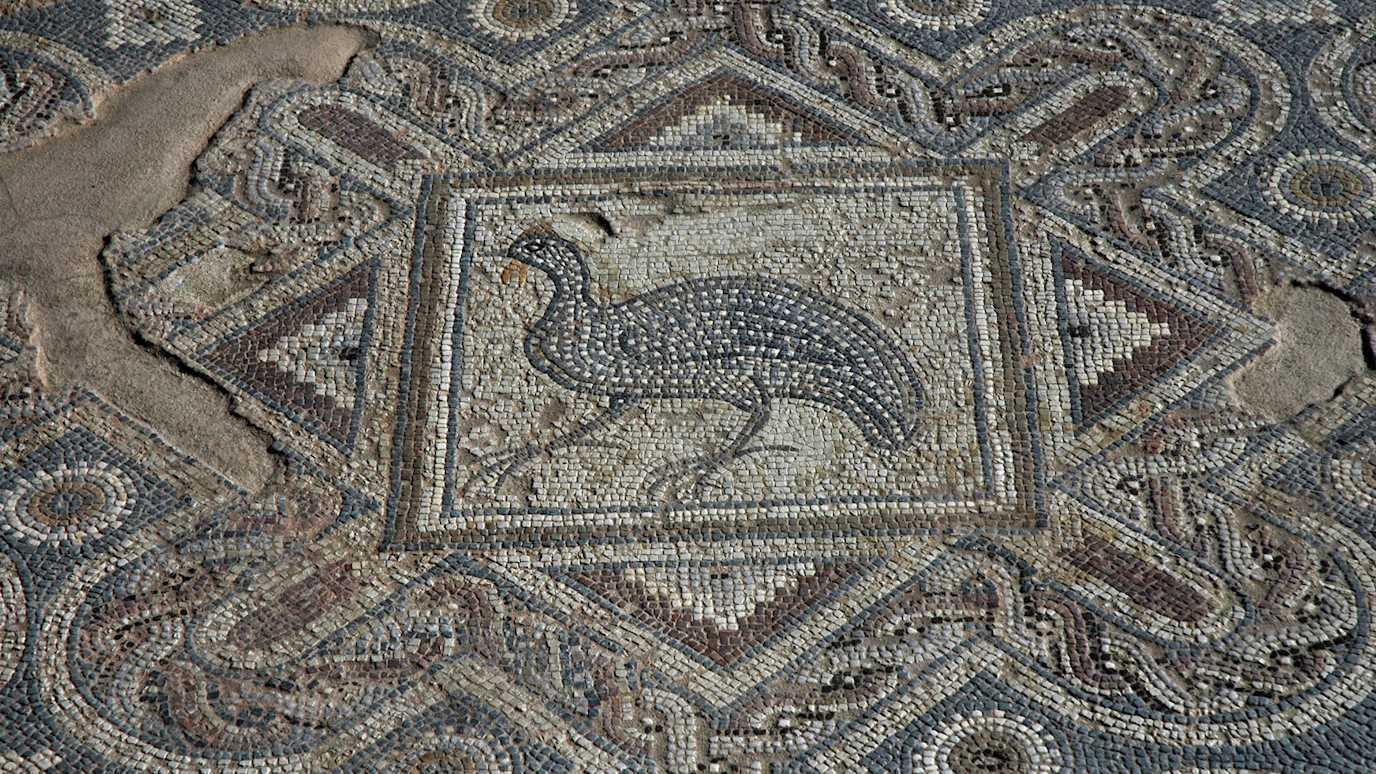Discover more about our 21 departments and schools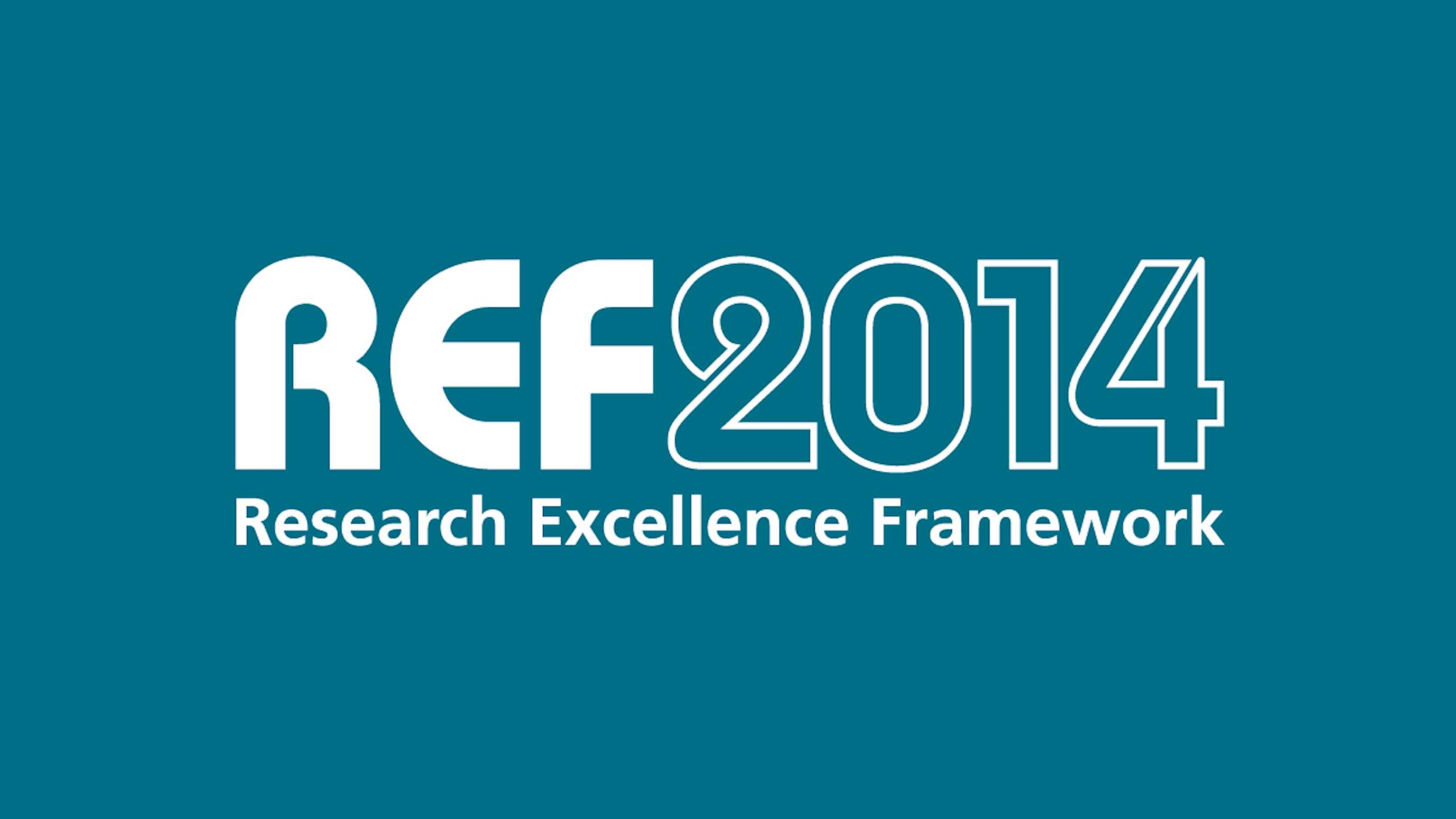Find out why Royal Holloway is in the top 25% of UK universities for research rated ‘world-leading’ or ‘internationally excellent’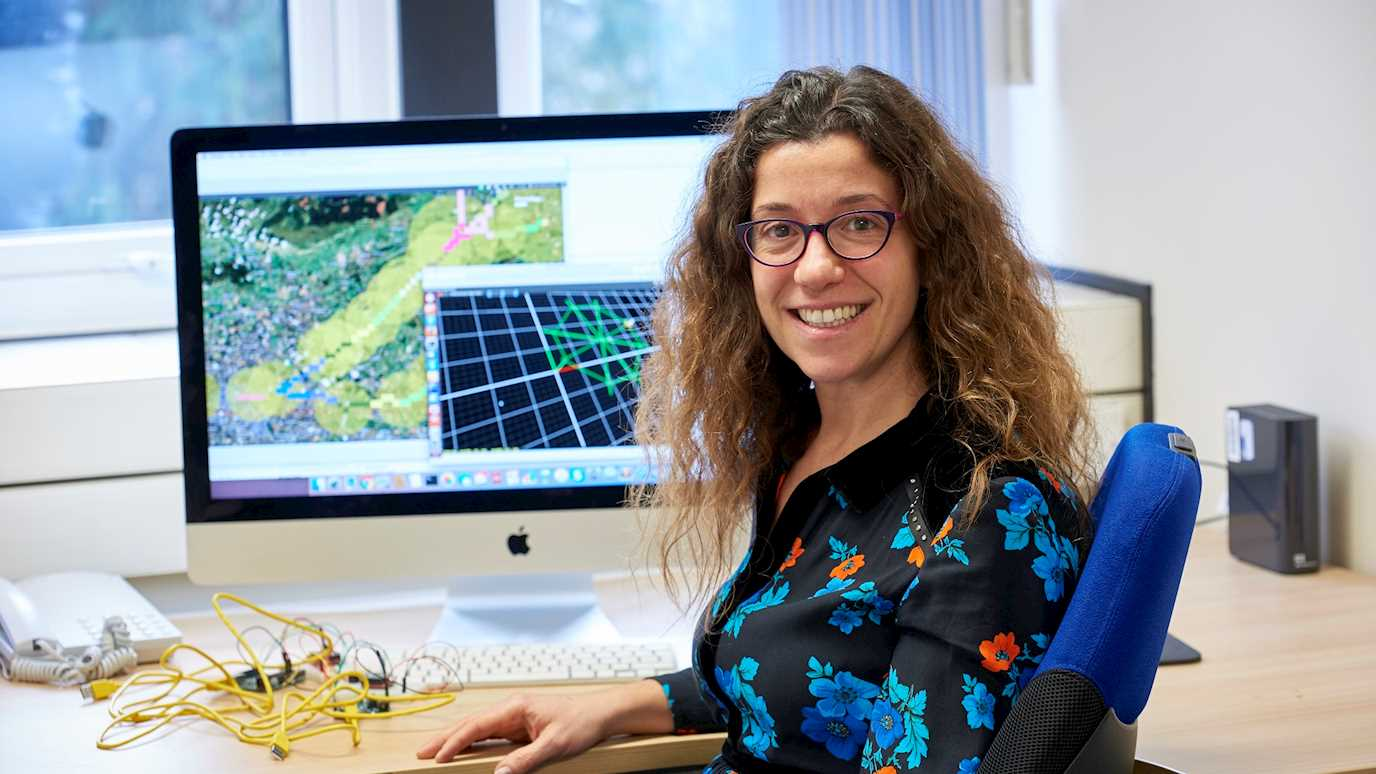They say the two most important days of your life are the day you were born, and the day you find out whyDiscover world-class research at Royal Holloway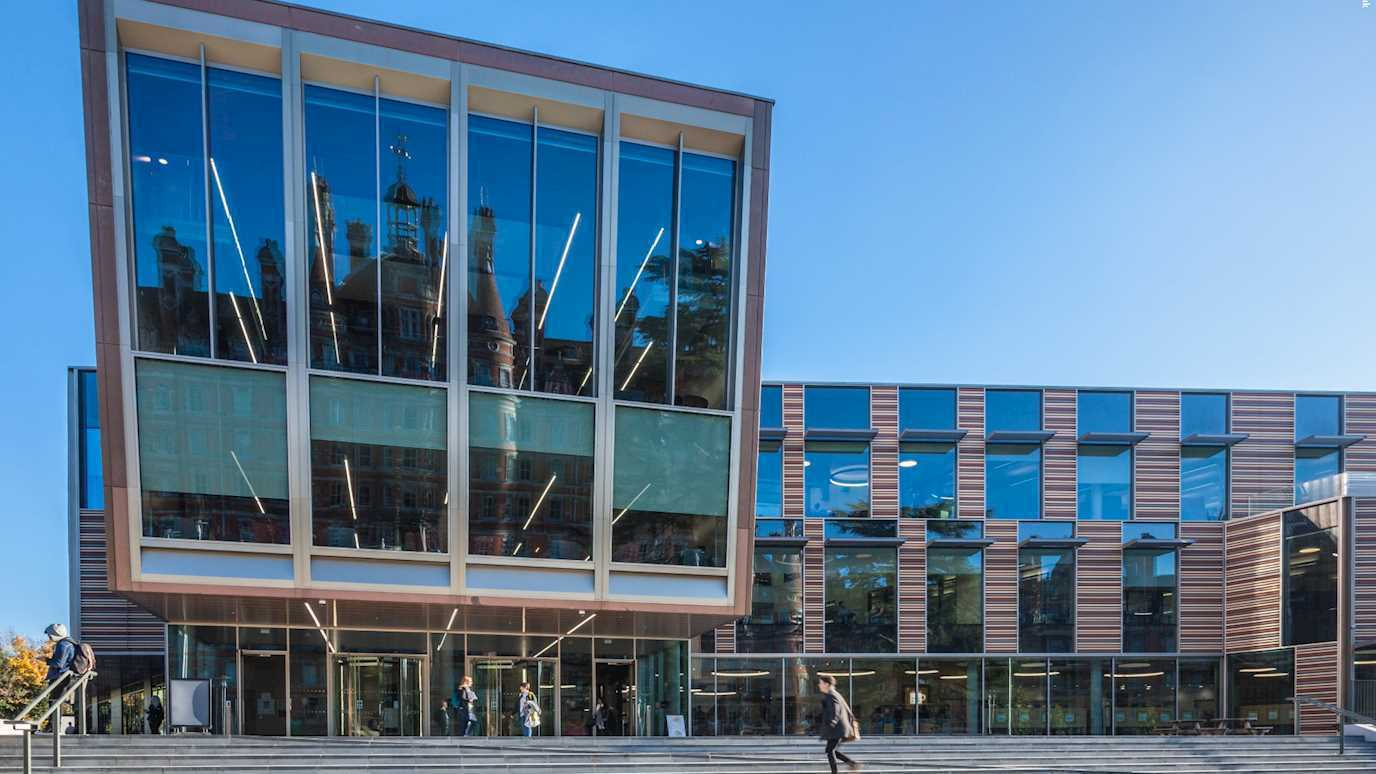Discover more about who we are today, and our vision for the futureRoyal Holloway began as two pioneering colleges for the education of women in the 19th century, and their spirit lives on todayWe’ve played a role in thousands of careers, some of them particularly remarkable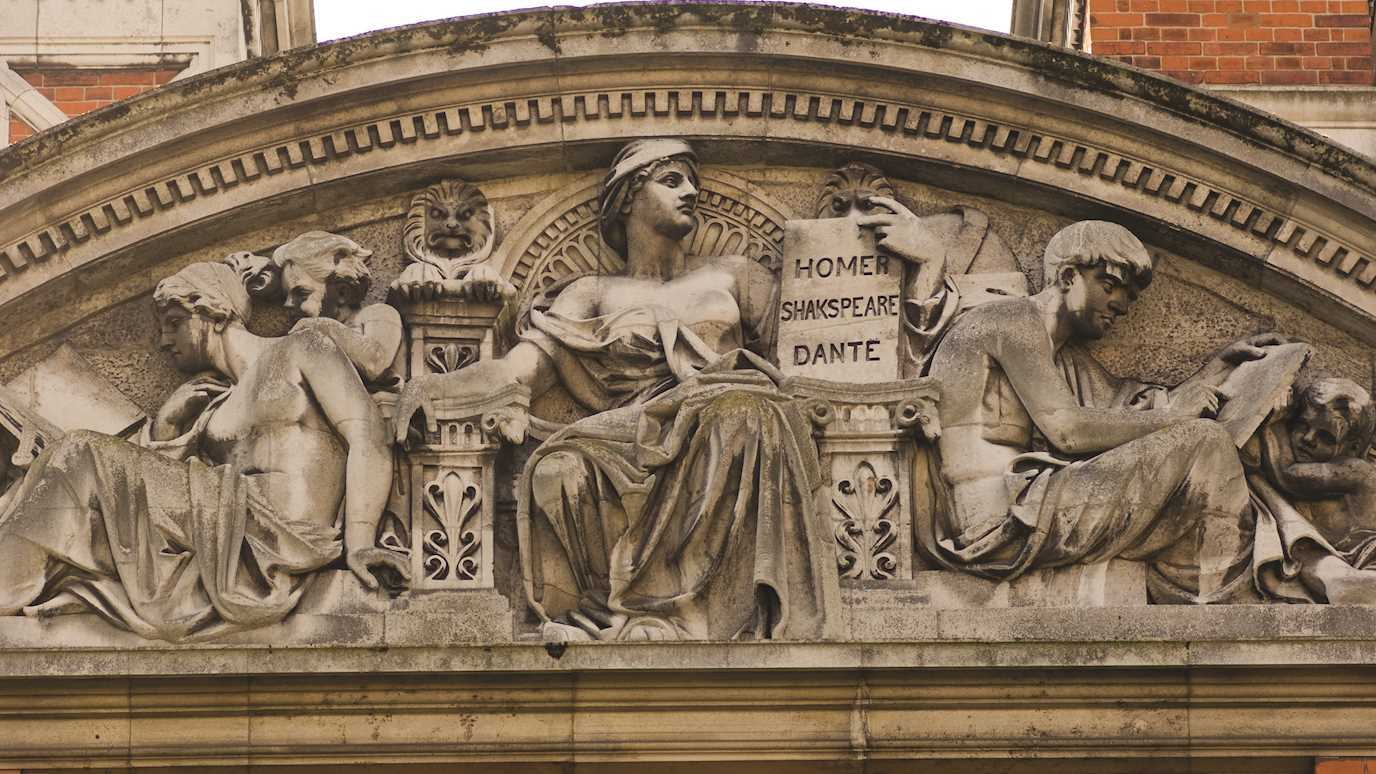Find about our decision-making processes and the people who lead and manage Royal Holloway today